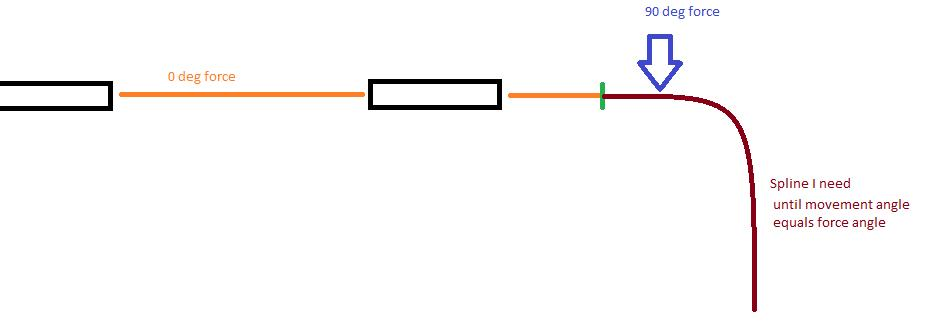# Forces acting at an angle

## Recommended Posts

Hello everyone,

I started programming motion calculation to get into math and physics a little deeper again.

I know that my problems have already been solved by other people around the globe every day, but I don't want to use the simple approach with a physics engine for now. I want to understand how things work.

So. Here is my problem: I have an entity with mass and velocity and it is currently heading 0. Now, I apply a side force (centripetal force). It might come from any angle.
My current implementation uses a standard centripetal force calculation using a circle, where I get the radius from mass and velocity and can tell where my new point is, given the amount of time that has passed between two frames.

Yet, in theory, that is not the correct calculation, if we delete the time aspect. Why? because my force direction is absolute. I am not in a car where I apply angle to wheels so I would go in a circle forever. I want to go e. g. to 90deg while currently, I am heading to 0. The centripetal force calculation with the circle only works approximately and while we assume that the framerate is high enough so we don't slip through the 90° point (because e. g. at 89, where the centripetal force amount is only 1deg, if enough time passes, the next point in the circle could be more than 90deg relative to the starting point where the force was initially applied). It could then potentially go back and forth a little.

So what I would need is a spline that is correct at the current time and then I can set the relative time that has passed and that gives me a new point where I am. Of course, when my angle changes, I need a new spline. But as long as no parameters are changed, I need to have a calculated spline on which I can move. But silly me, I did only do middle school and I have never been in touch with such calculations.

Can someone help me with that?
I hope my problem is clear. See the attached screenshot.## Join the conversation

You can post now and register later. If you have an account, sign in now to post with your account.
Note: Your post will require moderator approval before it will be visible.×   Pasted as rich text.   Paste as plain text instead

Only 75 emoji are allowed.